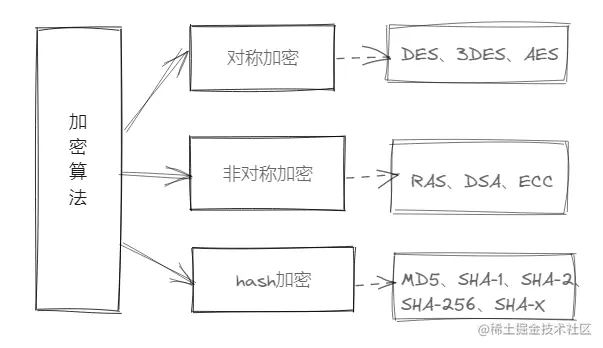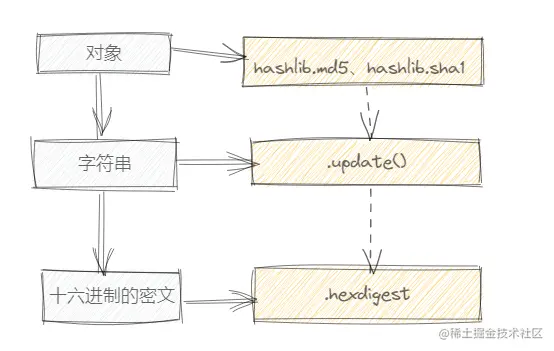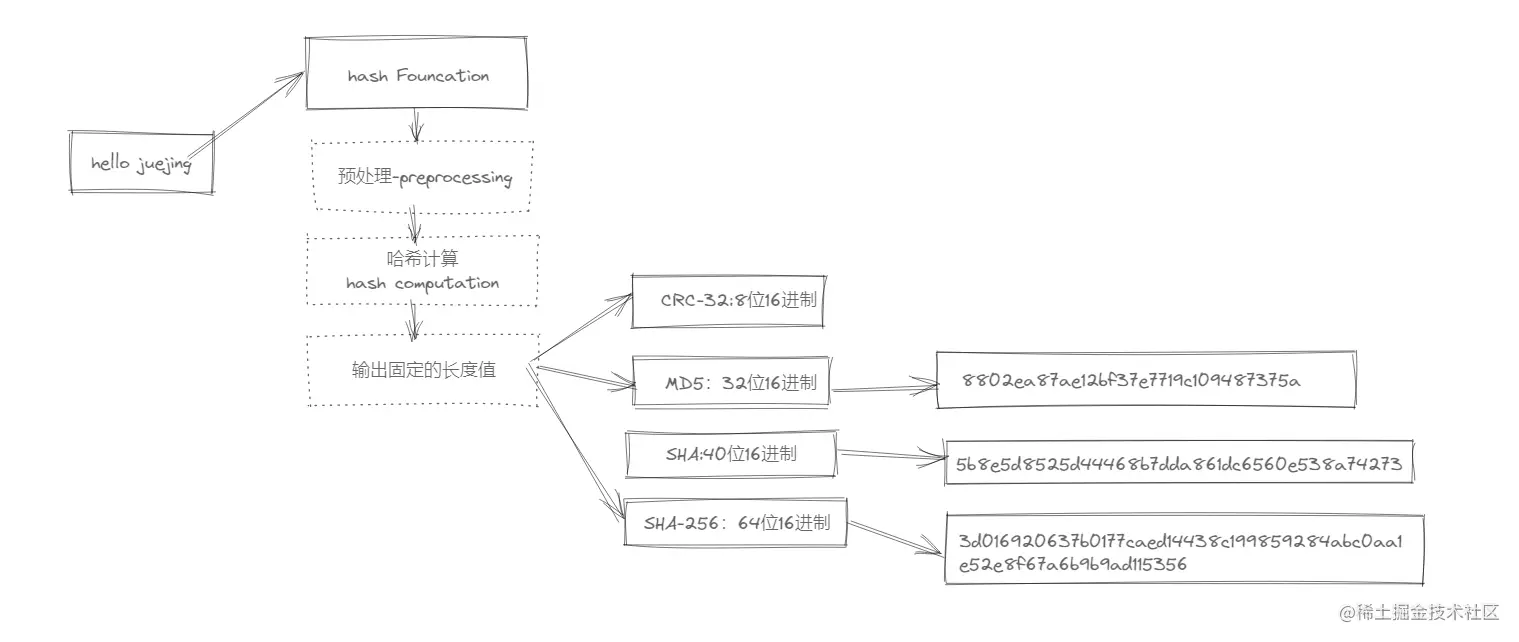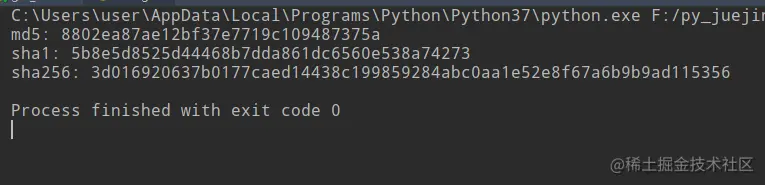# Python hashlib module

2022-01-31 01:08:58This is my participation 11 The fourth of the yuegengwen challenge 3 God , Check out the activity details ：2021 One last more challenge

# Preface

In the age of the Internet , We all need to be encrypted in the process of transmission in the network , The common encryption methods of network data transmission are MD5 and RSA Algorithm .

Of course , There are many encryption algorithms, according to encryption 、 Decryption methods are divided into ： Symmetric encryption 、 Asymmetric encryption 、hash encryption .among MD5 The basis of the algorithm is the use of hash Algorithm , For different security hash Security algorithms ,Python A common interface is also proposed hashlib modular .

In this issue , We come to the right Python Provides a general interface for secure hash algorithms -hashlib Module to learn ,Let's go ~

# 1. hashlib The module overview

hashlib yes Python Built in general interface module for security hashing and message summarization .hashlib Module support provides current mainstream services hash Algorithms such as MD5、SHA-1、SHA-2、SHA-256 And other general construction methods , And return a with the same interface hash object .

• ## hashlib Module features

• Provide mainstream hash Algorithm operation , replace md5 Module and sha modular
• Simple module call , No need to download and install
• It is mainly used in text encryption scenarios, such as user login authentication
• ## hashlib How to use the module

• Import hashlib library ：import hashlib
• Create an encrypted object
• Encrypt the string
• Get converted to new N individual Bit# 2. hashlib working principle

hashlib The encryption method in the module is common hash Algorithm .hash The algorithm is also called hash table （hash table）, Also known as hash table .

• ## hash Algorithm characteristics

• With unique certainty , Different strings are different after encryption
• Irreversibility ,hash Only encryption, no decryption process
• Hash collision , Output and input values are not one-to-one correspondence
• ## hash Construction method

• Direct addressing method

• Formula 1 ：hash(key) = key
• Formula 2 ：hash(key) = a*key+b
• We can see that the direct addressing method has the characteristics of linearity , Therefore, it is applicable in the case of continuous keyword distribution
• Mathematical analysis method

• The way ： Extract the random number bits in the keyword , Splice them into hash addresses
• Applicable scenario ： When the keyword is known , Analyze the value of each bit in the keyword
• Divide and leave remainder method

• The formula ：hash(key) = key % p
• The divisor adopts the operation of taking remainder modulus
• ## hash The algorithm process

• hash Function received the input string , First, we will preprocess - Hash computing - Enter summary
• Preprocessing ： Fill the string 、 Division N block , by hash To initialize
• Hash computing ： Complete the preprocessed data with the specified algorithm to generate a message summary
• Each one is specified hash The algorithm will only generate a fixed length summary , The longer the length, the higher the security• ## hash Commonly used algorithm

• ### MD5

MD5:message-Digest Algorithm 5 Information - Abstract algorithm 5, The algorithm is used to verify the integrity of information

• Calculation method ： Seeking remainder 、 Remainder 、 Adjust the length 、 Loop the linked variables to get the result

• purpose ： Mainly used for file verification

• ### SHA-1

SHA：secure Hash Algorithm Secure hash algorithm 1, Is a cryptographic hash algorithm ,SHA-1 Summary messages can be generated as 40 Bit 16 Base is 160 position （20 byte ） Hash value

• purpose ：TSL、SSL、PGP、SSH And other protocols

# 3. hashlib Attribute method

hashlib Module related attributes

attribute effect
hashlib.algorithms_guaranteed Ensure that hash algorithm names are supported on all platforms
hashlib.algorithms_available Ensure that the platform runs Python Available on the interpreter hash The name of the algorithm

hashlib Construct properties related to objects

attribute effect
hash.digest_size The size of the hash object in bytes
hash.block_size Represents the internal block size of the hash algorithm in bytes
hash.name The name of the hash object

hashlib Module related methods can support the mainstream at present hash Algorithm .

Method effect
hashlib.pbkdf2_hmac(hash_name,password,salt,itera,dklen=None) PKCS#5 Password based secret key derivation function 2, As HMAC As a pseudo-random function
hashlib.scrypt(password,*,salt) A cryptographic function derived from
hashlib.md5() md5 encryption
hashlib.sha1 sha1 encryption
hashlib.sha256 sha256 encryption
hashlib.blake2b () blake2b encryption
hashlib.blake2s black2s encryption

hashlib Construct object related methods

Method effect
hash.update(data) Represents the hash object in bytes
hash.digest() Returns the current passed to update() Method data summary
hash.hexdigest() With 16 The hexadecimal string represents the hash data value
hash.copy() take hash Object replication , Share a summary of the initial data

# 4. A profound

We learn hashlib The string is modified in the module hash Algorithm to deal with , Let's do it

``````import hashlib

text_md5 = hashlib.md5()
text_md5.update(bytes("hello juejing",encoding="utf-8"))
print("md5:",text_md5.hexdigest())

text_sha1 = hashlib.sha1()
text_sha1.update(bytes("hello juejing",encoding="utf-8"))
print("sha1:",text_sha1.hexdigest())

text_sha256 = hashlib.sha3_256()
text_sha256.update(bytes("hello juejing",encoding="utf-8"))
print("sha256:",text_sha256.hexdigest())

text_crc32 = hashlib.()
text_sha256.update(bytes("hello juejing",encoding="utf-8"))
print("sha256:",text_sha256.hexdigest())
Copy code ``````• ## Important note

• Add custom key Combined with string encryption （ Add salt ）
• With MD5 An example of encryption is as follows
• Add salt to write one ：
``````text_md5 = hashlib.md5(b"key")
text_md5.update(" character string ".encode("utf-8"))
Copy code ``````
• Add salt to write two ：
``````key = " character string "
yan = " character string 2"
text_md5 = hashlib.md5()
Copy code ``````

# summary

In this issue , We are right. hashlib Module hash Algorithm characteristics 、hashlib Module related expenditure algorithm for learning and practical operation .

stay hash In the algorithm, we often use md5/sha1/sha256 Mainly used for text verification 、 User login authentication and other data verification

meanwhile ,hash The algorithm ensures the integrity of the data , It is irreversible , At the same time, it will encounter violent collision , therefore hash The longer the length, the higher the security .

The above is the content of this issue , Welcome big guys to praise and comment , See you next time ~